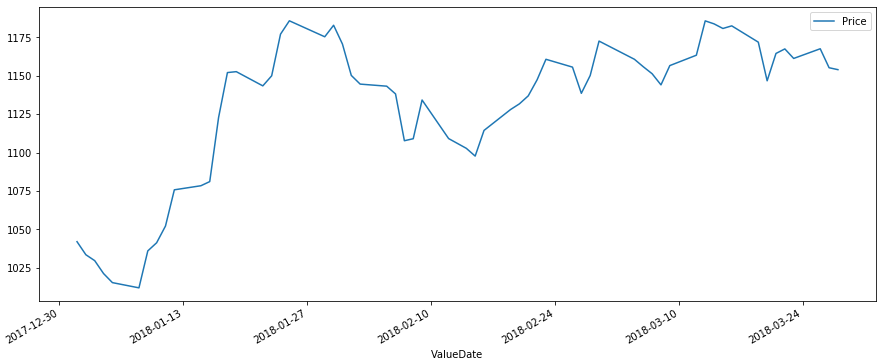# Python - Time Series

Time series is a series of data points in which each data point is associated with a timestamp. A simple example is the price of a stock in the stock market at different points of time on a given day. Another example is the amount of rainfall in a region at different months of the year.

In the below example we take the value of stock prices every day for a quarter for a particular stock symbol. We capture these values as a csv file and then organize them to a dataframe using pandas library. We then set the date field as index of the dataframe by recreating the additional Valuedate column as index and deleting the old valuedate column.

## Sample Data

Below is the sample data for the price of the stock on different days of a given quarter. The data is saved in a file named as stock.csv

```ValueDate	Price
01-01-2018,	1042.05
02-01-2018,	1033.55
03-01-2018,	1029.7
04-01-2018,	1021.3
05-01-2018,	1015.4
...
...
...
...
23-03-2018,	1161.3
26-03-2018,	1167.6
27-03-2018,	1155.25
28-03-2018,	1154
```

## Creating Time Series

```from datetime import datetime
import pandas as pd
import matplotlib.pyplot as plt

df = pd.DataFrame(data, columns = ['ValueDate', 'Price'])

# Set the Date as Index
df['ValueDate'] = pd.to_datetime(df['ValueDate'])
df.index = df['ValueDate']
del df['ValueDate']

df.plot(figsize=(15, 6))
plt.show()
```

Its output is as follows −## Useful Video Courses

Video

#### Python Online Training

187 Lectures 17.5 hours

Video

#### Python Essentials Online Training

55 Lectures 8 hours

Video

#### Learn Python Programming in 100 Easy Steps

136 Lectures 11 hours

Video

#### Python with Data Science

Best Seller

75 Lectures 13 hours

Video

#### Python 3 from scratch to become a developer in demand

Best Seller

70 Lectures 8.5 hours

Video

#### Python Data Science basics with Numpy, Pandas and Matplotlib

Most Popular

63 Lectures 6 hours